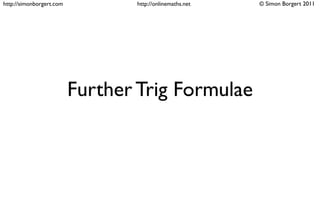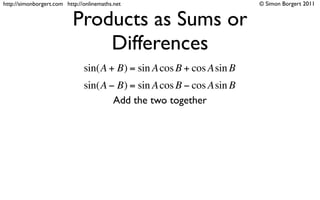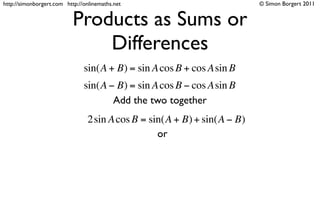Successfully reported this slideshow.

# Trig products as sum and differecnes

0

Share×
1 of 34
1 of 34

# Trig products as sum and differecnes

0

Share

Presentation that explains how the product to sum and sum to product formula's are developed.

Presentation that explains how the product to sum and sum to product formula's are developed.

## More Related Content

### Related Books

Free with a 14 day trial from Scribd

See all

### Related Audiobooks

Free with a 14 day trial from Scribd

See all

### Trig products as sum and differecnes

1. 1. http://simonborgert.com http://onlinemaths.net © Simon Borgert 2011 Further Trig Formulae
2. 2. http://simonborgert.com http://onlinemaths.net © Simon Borgert 2011 Products as Sums or Differences sin(A + B) = sin A cos B + cos Asin B sin(A − B) = sin A cos B − cos Asin B Add the two together
3. 3. http://simonborgert.com http://onlinemaths.net © Simon Borgert 2011 Products as Sums or Differences sin(A + B) = sin A cos B + cos Asin B sin(A − B) = sin A cos B − cos Asin B Add the two together 2sin A cos B = sin(A + B) + sin(A − B) or
4. 4. http://simonborgert.com http://onlinemaths.net © Simon Borgert 2011 Products as Sums or Differences sin(A + B) = sin A cos B + cos Asin B sin(A − B) = sin A cos B − cos Asin B Add the two together 2sin A cos B = sin(A + B) + sin(A − B) or 2sin A cos B = sin(sum) + sin(difference)
5. 5. http://simonborgert.com http://onlinemaths.net © Simon Borgert 2011 Products as Sums or Differences sin(A + B) = sin A cos B + cos Asin B sin(A − B) = sin A cos B − cos Asin B Add the two together 2sin A cos B = sin(A + B) + sin(A − B) or 2sin A cos B = sin(sum) + sin(difference) 1 1 sin A cos B = sin(sum) + sin(difference) 2 2
6. 6. http://simonborgert.com http://onlinemaths.net © Simon Borgert 2011 Products as Sums or Differences sin(A + B) = sin A cos B + cos Asin B sin(A − B) = sin A cos B − cos Asin B Subtract the second from the ﬁrst 2 cos Asin B = sin(A + B) − sin(A − B) or 2 cos Asin B = sin(sum) − sin(difference) 1 1 cos Asin B = sin(sum) − sin(difference) 2 2
7. 7. http://simonborgert.com http://onlinemaths.net © Simon Borgert 2011 Products as Sums or Differences cos(A + B) = cos A cos B − sin Asin B cos(A − B) = cos A cos B + sin Asin B Add the two together 2 cos A cos B = cos(A + B) + cos(A − B) or 2 cos A cos B = cos(sum) + cos(difference) 1 1 cos A cos B = cos(sum) + cos(difference) 2 2
8. 8. http://simonborgert.com http://onlinemaths.net © Simon Borgert 2011 Products as Sums or Differences cos(A + B) = cos A cos B − sin Asin B cos(A − B) = cos A cos B + sin Asin B Subtract the ﬁrst from the second 2sin Asin B = cos(A − B) − cos(A + B) or 2sin Asin B = cos(difference) − cos(sum) 1 1 sin Asin B = cos(difference) − cos(sum) 2 2
9. 9. http://simonborgert.com http://onlinemaths.net © Simon Borgert 2011 Summary 2sin A cos B = sin(sum) + sin(difference) 2 cos Asin B = sin(sum) − sin(difference) 2 cos A cos B = cos(sum) + cos(difference) 2sin Asin B = cos(difference) − cos(sum)
10. 10. http://simonborgert.com http://onlinemaths.net © Simon Borgert 2011 or... 1 1 sin A cos B = sin(sum) + sin(difference) 2 2 1 1 cos Asin B = sin(sum) − sin(difference) 2 2 1 1 cos A cos B = cos(sum) + cos(difference) 2 2 1 1 sin Asin B = cos(difference) − cos(sum) 2 2
11. 11. http://simonborgert.com http://onlinemaths.net © Simon Borgert 2011 Example 1 Express the product of cos 5x sin 3x as a sum of trigonometric functions
12. 12. http://simonborgert.com http://onlinemaths.net © Simon Borgert 2011 Example 1 Express the product of cos 5x sin 3x as a sum of trigonometric functions Step 1: Identify the product to sum formula
13. 13. http://simonborgert.com http://onlinemaths.net © Simon Borgert 2011 Example 1 Express the product of cos 5x sin 3x as a sum of trigonometric functions Step 1: Identify the product to sum formula 1 1 cos Asin B = sin(sum) − sin(difference) 2 2
14. 14. http://simonborgert.com http://onlinemaths.net © Simon Borgert 2011 Example 1 Express the product of cos 5x sin 3x as a sum of trigonometric functions Step 1: Identify the product to sum formula 1 1 cos Asin B = sin(sum) − sin(difference) 2 2 Step 2: Apply it to the given product
15. 15. http://simonborgert.com http://onlinemaths.net © Simon Borgert 2011 Example 1 Express the product of cos 5x sin 3x as a sum of trigonometric functions Step 1: Identify the product to sum formula 1 1 cos Asin B = sin(sum) − sin(difference) 2 2 Step 2: Apply it to the given product 1 1 cos 5x sin 3x = sin(5x + 3x) − sin(5x − 3x) 2 2
16. 16. http://simonborgert.com http://onlinemaths.net © Simon Borgert 2011 Example 1 Express the product of cos 5x sin 3x as a sum of trigonometric functions Step 1: Identify the product to sum formula 1 1 cos Asin B = sin(sum) − sin(difference) 2 2 Step 2: Apply it to the given product 1 1 cos 5x sin 3x = sin(5x + 3x) − sin(5x − 3x) 2 2 1 1 = sin(8x) − sin(2x) 2 2
17. 17. http://simonborgert.com http://onlinemaths.net © Simon Borgert 2011 Example 2 Express the product of 2sin3x sin 2x as a difference of trigonometric functions Step 1: Identify the product to sum formula
18. 18. http://simonborgert.com http://onlinemaths.net © Simon Borgert 2011 Example 2 Express the product of 2sin3x sin 2x as a difference of trigonometric functions Step 1: Identify the product to sum formula 2sin Asin B = cos(difference) − cos(sum)
19. 19. http://simonborgert.com http://onlinemaths.net © Simon Borgert 2011 Example 2 Express the product of 2sin3x sin 2x as a difference of trigonometric functions Step 1: Identify the product to sum formula 2sin Asin B = cos(difference) − cos(sum) Step 2: Apply it to the given product
20. 20. http://simonborgert.com http://onlinemaths.net © Simon Borgert 2011 Example 2 Express the product of 2sin3x sin 2x as a difference of trigonometric functions Step 1: Identify the product to sum formula 2sin Asin B = cos(difference) − cos(sum) Step 2: Apply it to the given product 2sin 3x sin 2x = cos(3x − 2x) − cos(3x + 2x)
21. 21. http://simonborgert.com http://onlinemaths.net © Simon Borgert 2011 Example 2 Express the product of 2sin3x sin 2x as a difference of trigonometric functions Step 1: Identify the product to sum formula 2sin Asin B = cos(difference) − cos(sum) Step 2: Apply it to the given product 2sin 3x sin 2x = cos(3x − 2x) − cos(3x + 2x) = cos x − cos 5x
22. 22. http://simonborgert.com http://onlinemaths.net © Simon Borgert 2011 Sums and Differences as Products 2sin A cos B = sin(A + B) + sin(A − B) 2 cos Asin B = sin(A + B) − sin(A − B) 2 cos A cos B = cos(A + B) + cos(A − B) 2sin Asin B = cos(A − B) − cos(A + B)
23. 23. http://simonborgert.com http://onlinemaths.net © Simon Borgert 2011 Sums and Differences as Products Let U = A + B and V = A − B 2sin A cos B = sin(A + B) + sin(A − B) U +V U −V 2 cos Asin B = sin(A + B) − sin(A − B) ∴A = and B = 2 cos A cos B = cos(A + B) + cos(A − B) 2 2 2sin Asin B = cos(A − B) − cos(A + B)
24. 24. http://simonborgert.com http://onlinemaths.net © Simon Borgert 2011 Sums and Differences as Products Let U = A + B and V = A − B 2sin A cos B = sin(A + B) + sin(A − B) U +V U −V 2 cos Asin B = sin(A + B) − sin(A − B) ∴A = and B = 2 cos A cos B = cos(A + B) + cos(A − B) 2 2 2sin Asin B = cos(A − B) − cos(A + B)
25. 25. http://simonborgert.com http://onlinemaths.net © Simon Borgert 2011 Sums and Differences as Products Let U = A + B and V = A − B 2sin A cos B = sin(A + B) + sin(A − B) U +V U −V 2 cos Asin B = sin(A + B) − sin(A − B) ∴A = and B = 2 cos A cos B = cos(A + B) + cos(A − B) 2 2 2sin Asin B = cos(A − B) − cos(A + B) so the formulas become
26. 26. http://simonborgert.com http://onlinemaths.net © Simon Borgert 2011 Sums and Differences as Products Let U = A + B and V = A − B 2sin A cos B = sin(A + B) + sin(A − B) U +V U −V 2 cos Asin B = sin(A + B) − sin(A − B) ∴A = and B = 2 cos A cos B = cos(A + B) + cos(A − B) 2 2 U +V  U −V  sinU + sinV = 2sin   cos  2sin Asin B = cos(A − B) − cos(A + B)  2   2   U +V  U −V  sinU − sinV = 2 cos   sin   2   2   U +V  U −V  cosU + cosV = 2 cos   cos  so the formulas become  2   2   U +V  U −V  cosV − cosU = 2sin   sin   2   2   U +V  U −V  cosU − cosV = −2sin   sin   2   2  
27. 27. http://simonborgert.com http://onlinemaths.net © Simon Borgert 2011 Sums and Differences as Products U +V  U −V  sinU + sinV = 2sin   cos   1  1   2   2  sin+ sin = 2sin  sum  cos  difference 2  2  U +V  U −V  sinU − sinV = 2 cos   sin   2   2   1  1  sin− sin = 2 cos  sum  sin  difference 2  2  U +V  U −V  cosU + cosV = 2 cos   cos   2   2   1  1  cos+ cos = 2 cos  sum  cos  difference 2  2  U +V  U −V  cosV − cosU = 2sin   sin   1  1   2   2  cos− cos = −2sin  sum  sin  difference 2  2  U +V  U −V  cosU − cosV = −2sin   sin    2   2 
28. 28. http://simonborgert.com http://onlinemaths.net © Simon Borgert 2011 Example 3 Express cos 3x + cos 7x as a product
29. 29. http://simonborgert.com http://onlinemaths.net © Simon Borgert 2011 Example 3 Express cos 3x + cos 7x as a product Step 1: Identify the sum to product formula
30. 30. http://simonborgert.com http://onlinemaths.net © Simon Borgert 2011 Example 3 Express cos 3x + cos 7x as a product Step 1: Identify the sum to product formula 1  1  cos+ cos = 2 cos  sum  cos  difference 2  2 
31. 31. http://simonborgert.com http://onlinemaths.net © Simon Borgert 2011 Example 3 Express cos 3x + cos 7x as a product Step 1: Identify the sum to product formula 1  1  cos+ cos = 2 cos  sum  cos  difference 2  2  Step 2: Apply it to the given product
32. 32. http://simonborgert.com http://onlinemaths.net © Simon Borgert 2011 Example 3 Express cos 3x + cos 7x as a product Step 1: Identify the sum to product formula 1  1  cos+ cos = 2 cos  sum  cos  difference 2  2  Step 2: Apply it to the given product 1  1  cos 3x + cos 7x = 2 cos  (3x + 7x) cos  (3x − 7x) 2  2 
33. 33. http://simonborgert.com http://onlinemaths.net © Simon Borgert 2011 Example 3 Express cos 3x + cos 7x as a product Step 1: Identify the sum to product formula 1  1  cos+ cos = 2 cos  sum  cos  difference 2  2  Step 2: Apply it to the given product 1  1  cos 3x + cos 7x = 2 cos  (3x + 7x) cos  (3x − 7x) 2  2  = 2 cos ( 5x ) cos ( −4x )
34. 34. http://simonborgert.com http://onlinemaths.net © Simon Borgert 2011 Example 3 Express cos 3x + cos 7x as a product Step 1: Identify the sum to product formula 1  1  cos+ cos = 2 cos  sum  cos  difference 2  2  Step 2: Apply it to the given product 1  1  cos 3x + cos 7x = 2 cos  (3x + 7x) cos  (3x − 7x) 2  2  = 2 cos ( 5x ) cos ( −4x ) = 2 cos ( 5x ) cos ( 4x ) as cos(−x) = cos x

• \n
• \n
• \n
• \n
• \n
• \n
• \n
• \n
• \n
• \n
• \n
• \n
• \n
• \n
• \n
• \n
• \n
• \n
• \n
• \n
• \n
• \n
• \n
• \n
• \n
• \n
• \n
• \n
• \n
• \n
• \n
• \n
• \n
• \n